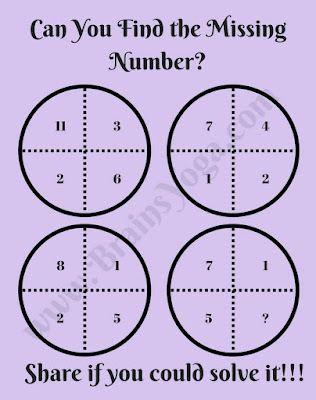Here is the Maths Missing Number Picture puzzle. Here four number are given inside the Circle in different segments. These numbers are related to each other Mathematically. Find this Maths pattern or relations among the number and then find the missing number which will replace the question mark.Can you find the missing number?